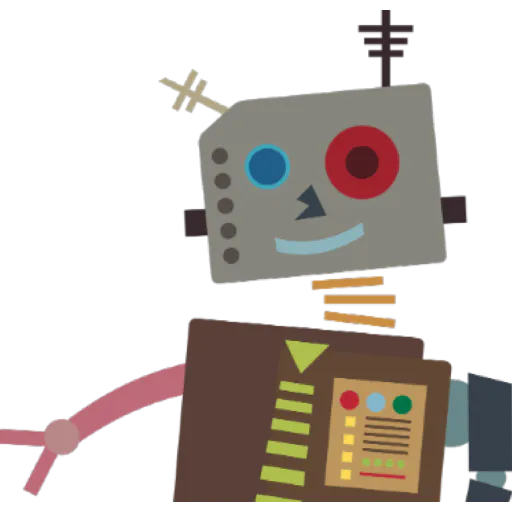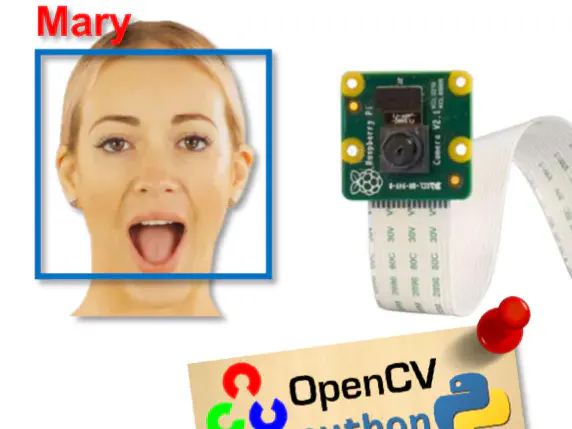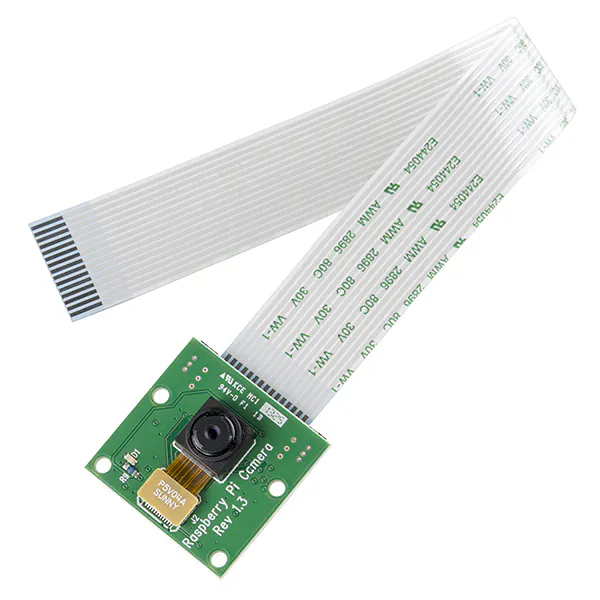# Real-Time Face Recognition: An End-to-End Project

We will learn step by step, how to use a PiCam to recognize faces in real-time.

IntermediateFull instructions provided12 hours227,589## Things used in this project

### Hardware componentsRaspberry Pi 3 Model B
×1Raspberry Pi Camera Module
×1

### Software apps and online servicesOpenCV
 Python 3

## Code

### Teste Camera

Python
```import numpy as np
import cv2

cap = cv2.VideoCapture(0)
cap.set(3,640) # set Width
cap.set(4,480) # set Height

while(True):
frame = cv2.flip(frame, -1) # Flip camera vertically
gray = cv2.cvtColor(frame, cv2.COLOR_BGR2GRAY)

cv2.imshow('frame', frame)
cv2.imshow('gray', gray)

k = cv2.waitKey(30) & 0xff
if k == 27: # press 'ESC' to quit
break

cap.release()
cv2.destroyAllWindows()
```

### Face Detection

Python
```import numpy as np
import cv2

cap = cv2.VideoCapture(0)
cap.set(3,640) # set Width
cap.set(4,480) # set Height

while True:
img = cv2.flip(img, -1)
gray = cv2.cvtColor(img, cv2.COLOR_BGR2GRAY)
gray,
scaleFactor=1.2,
minNeighbors=5,
minSize=(20, 20)
)

for (x,y,w,h) in faces:
cv2.rectangle(img,(x,y),(x+w,y+h),(255,0,0),2)
roi_gray = gray[y:y+h, x:x+w]
roi_color = img[y:y+h, x:x+w]

cv2.imshow('video',img)

k = cv2.waitKey(30) & 0xff
if k == 27: # press 'ESC' to quit
break

cap.release()
cv2.destroyAllWindows()
```

### Data Gatering

Python
```import cv2
import os

cam = cv2.VideoCapture(0)
cam.set(3, 640) # set video width
cam.set(4, 480) # set video height

# For each person, enter one numeric face id
face_id = input('\n enter user id end press <return> ==>  ')

print("\n [INFO] Initializing face capture. Look the camera and wait ...")
# Initialize individual sampling face count
count = 0

while(True):
img = cv2.flip(img, -1) # flip video image vertically
gray = cv2.cvtColor(img, cv2.COLOR_BGR2GRAY)
faces = face_detector.detectMultiScale(gray, 1.3, 5)

for (x,y,w,h) in faces:
cv2.rectangle(img, (x,y), (x+w,y+h), (255,0,0), 2)
count += 1

# Save the captured image into the datasets folder
cv2.imwrite("dataset/User." + str(face_id) + '.' + str(count) + ".jpg", gray[y:y+h,x:x+w])

cv2.imshow('image', img)

k = cv2.waitKey(100) & 0xff # Press 'ESC' for exiting video
if k == 27:
break
elif count >= 30: # Take 30 face sample and stop video
break

# Do a bit of cleanup
print("\n [INFO] Exiting Program and cleanup stuff")
cam.release()
cv2.destroyAllWindows()
```

### Trainer

Python
```import cv2
import numpy as np
from PIL import Image
import os

# Path for face image database
path = 'dataset'

recognizer = cv2.face.LBPHFaceRecognizer_create()

# function to get the images and label data
def getImagesAndLabels(path):
imagePaths = [os.path.join(path,f) for f in os.listdir(path)]
faceSamples=[]
ids = []
for imagePath in imagePaths:
PIL_img = Image.open(imagePath).convert('L') # convert it to grayscale
img_numpy = np.array(PIL_img,'uint8')
id = int(os.path.split(imagePath)[-1].split("."))
faces = detector.detectMultiScale(img_numpy)
for (x,y,w,h) in faces:
faceSamples.append(img_numpy[y:y+h,x:x+w])
ids.append(id)
return faceSamples,ids

print ("\n [INFO] Training faces. It will take a few seconds. Wait ...")
faces,ids = getImagesAndLabels(path)
recognizer.train(faces, np.array(ids))

# Save the model into trainer/trainer.yml
recognizer.write('trainer/trainer.yml') # recognizer.save() worked on Mac, but not on Pi

# Print the numer of faces trained and end program
print("\n [INFO] {0} faces trained. Exiting Program".format(len(np.unique(ids))))
```

### Recognizer

Python
```import cv2
import numpy as np
import os

recognizer = cv2.face.LBPHFaceRecognizer_create()

font = cv2.FONT_HERSHEY_SIMPLEX

#iniciate id counter
id = 0

# names related to ids: example ==> Marcelo: id=1,  etc
names = ['None', 'Marcelo', 'Paula', 'Ilza', 'Z', 'W']

# Initialize and start realtime video capture
cam = cv2.VideoCapture(0)
cam.set(3, 640) # set video widht
cam.set(4, 480) # set video height

# Define min window size to be recognized as a face
minW = 0.1*cam.get(3)
minH = 0.1*cam.get(4)

while True:
img = cv2.flip(img, -1) # Flip vertically
gray = cv2.cvtColor(img,cv2.COLOR_BGR2GRAY)

gray,
scaleFactor = 1.2,
minNeighbors = 5,
minSize = (int(minW), int(minH)),
)

for(x,y,w,h) in faces:
cv2.rectangle(img, (x,y), (x+w,y+h), (0,255,0), 2)
id, confidence = recognizer.predict(gray[y:y+h,x:x+w])

# Check if confidence is less them 100 ==> "0" is perfect match
if (confidence < 100):
id = names[id]
confidence = "  {0}%".format(round(100 - confidence))
else:
id = "unknown"
confidence = "  {0}%".format(round(100 - confidence))

cv2.putText(img, str(id), (x+5,y-5), font, 1, (255,255,255), 2)
cv2.putText(img, str(confidence), (x+5,y+h-5), font, 1, (255,255,0), 1)

cv2.imshow('camera',img)

k = cv2.waitKey(10) & 0xff # Press 'ESC' for exiting video
if k == 27:
break

# Do a bit of cleanup
print("\n [INFO] Exiting Program and cleanup stuff")
cam.release()
cv2.destroyAllWindows()
```

### Code snippet #11

Plain text
```faces = faceCascade.detectMultiScale(
gray,
scaleFactor=1.2,
minNeighbors=5,
minSize=(20, 20)
)
```

### Github file

https://github.com/Mjrovai/OpenCV-Object-Face-Tracking/blob/master/simpleCamTest.py